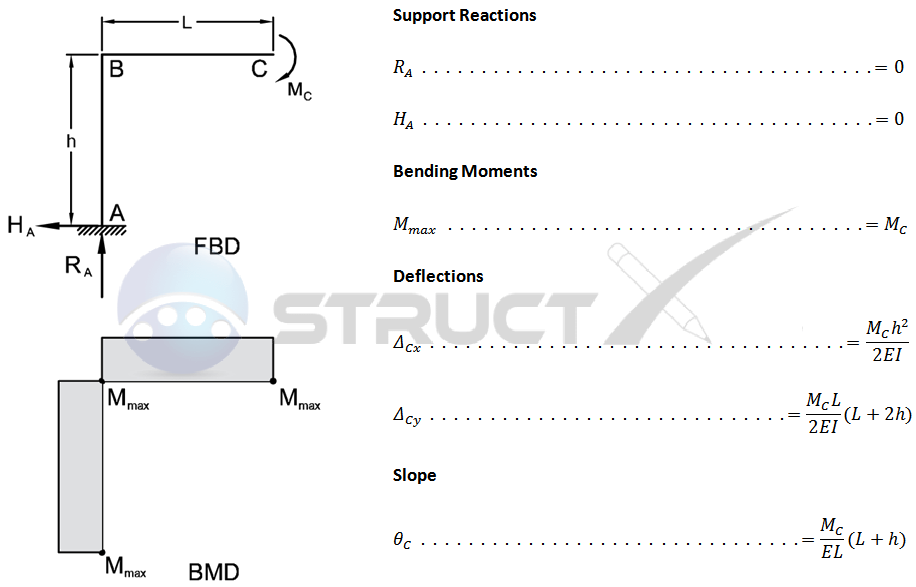# Two Member Frame (Fixed / Free) - Free End Bending MomentMore Frames# Notation and Units

## Metric and Imperial Units

The above frame formulas may be used with both imperial and metric units. As with all calculations care must be taken to keep consistent units throughout with examples of units which should be adopted listed below:

# Notation

• FBD = free body diagram
• BMD = bending moment diagram

• A, B & C = points of interest on frame
• h = height of frame, in or mm
• H = horizontal reaction load at bearing point, lbf or N
• E = modulus of elasticity, psi or MPa
• Ih = horizontal member second moment of area, in4 or mm4
• Iv = vertical member second moment of area, in4 or mm4
• L = span length under consideration, in or mm
• M = maximum bending moment, lbf.in or Nmm
• R = vertical reaction load at bearing point, lbf or N
• ∆ = deflection or deformation, in or mm